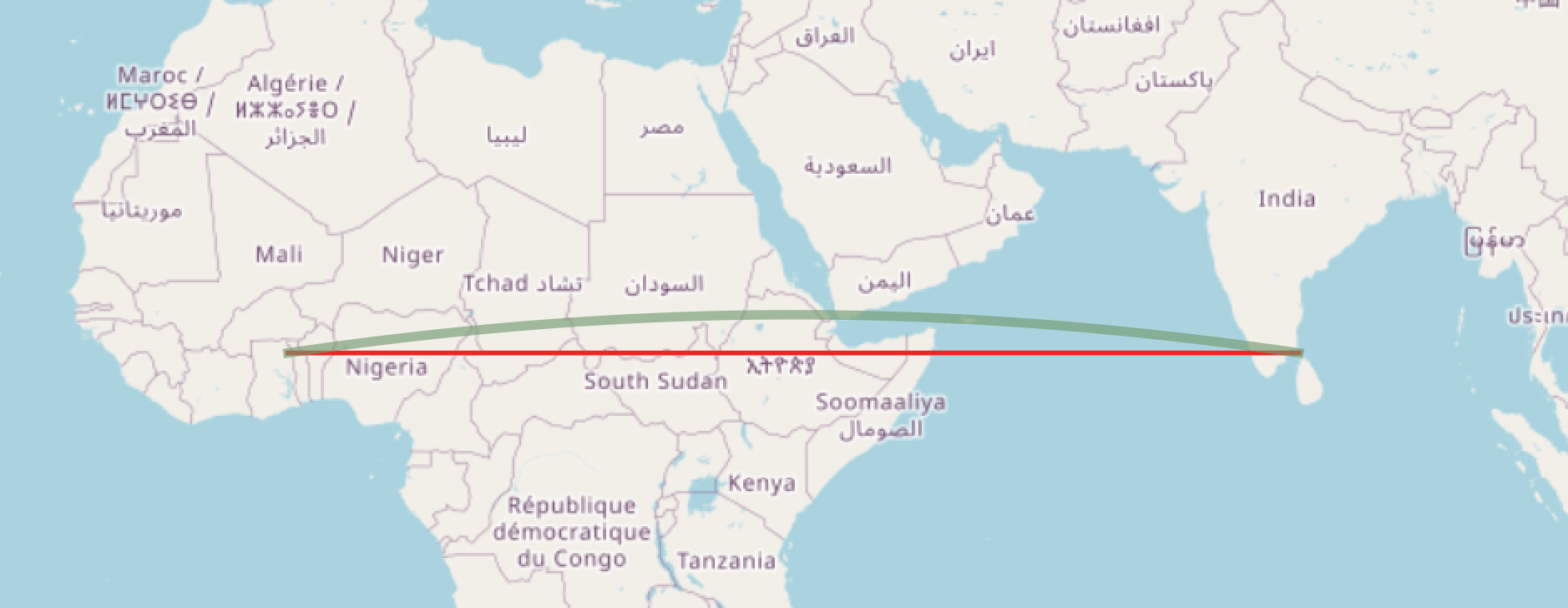# Why is the geography intersects function wrong?

Firstly, it’s not! But a frequent question we get is of the form, “the geography intersects test is saying this point is side this box, when clearly it is not, why?”

Here’s the example:

``````WITH wkt AS (
SELECT
'POLYGON((0 0, 80 0, 80 10, 0 10, 0 0))' AS box,
'POINT(40 10.1)' AS pt
)
SELECT
ST_Intersects(wkt.box::geography, wkt.pt::geography) AS intersects_geog,
ST_Intersects(wkt.box::geometry, wkt.pt::geometry) AS intersects_geom
FROM wkt;
``````

Same box, same point, but different answers depending on if you evaluate as geometry, or geography. Why?

`````` intersects_geog | intersects_geom
-----------------+-----------------
t               | f
``````

The answer is in the different spaces the two types work within:

• geometry is shapes placed on a Cartesian plane (or volume, if you add a third dimension) and the shortest path between any two points is a straight line.
• geography is shapes placed on the surface of a sphere and the shortest path between any two points is a great circle.

In our example, the northernmost edge of the box will be a `LINESTRING(0 10, 80 10)` which in geometry is a horizontal straight line, but in geography is a great circle that curves slightly to the north, so much so that it passes to the north of our test ‘POINT(40 10.1)’.In reasoning about geography, it is always important to remember that the edges are great circles. East/west lines north of the equator will bend north a little, and south of the equator will bend south a little.